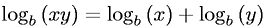Equations > Algebra > Logarithms > Sum of Logarithms Property

### Sum of Logarithms PropertyLatex Code:

MathML Code:

 ${\mathrm{log}}_{b}\left(\mathrm{xy}\right)={\mathrm{log}}_{b}\left(x\right)+{\mathrm{log}}_{b}\left(y\right)$

MathType 5.0: